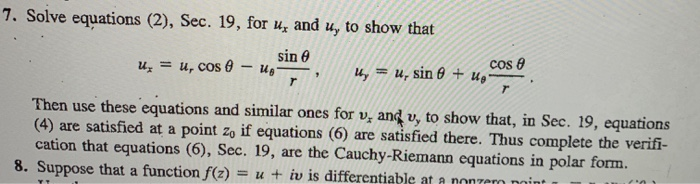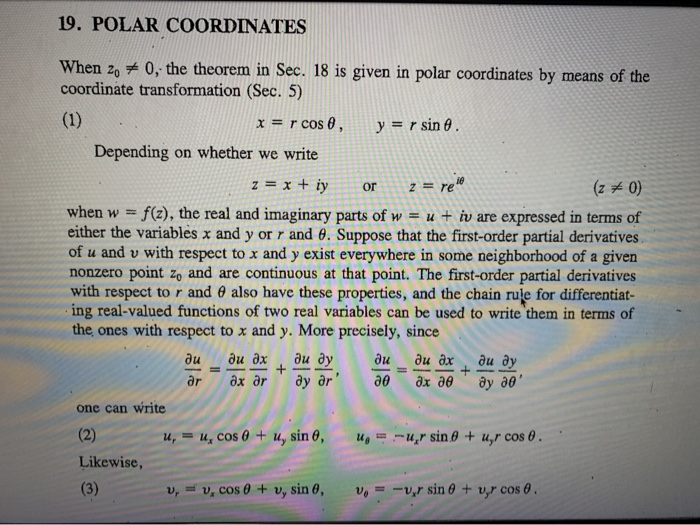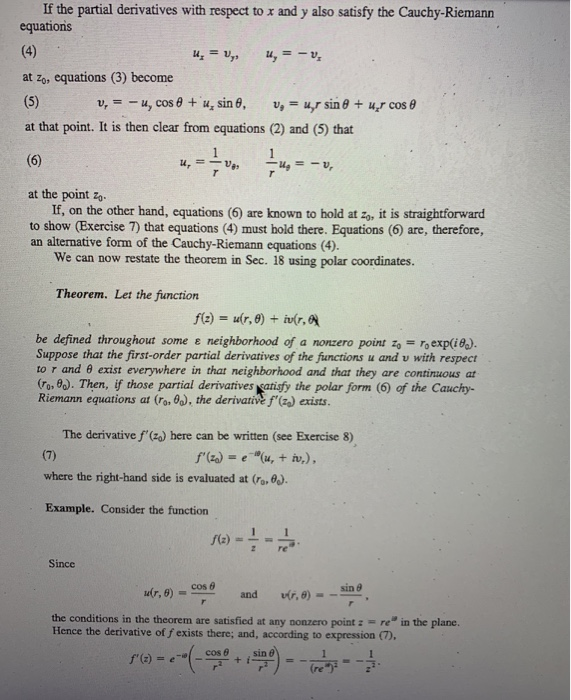# Question Solved1 Answer7. Solve equations (2), Sec. 19, for Uy and uy to show that sin e U = , cos 0 - U Hy = u, sin 0 + un cos e T T Then use these equations and similar ones for v, and v, to show that, in Sec. 19, equations (4) are satisfied at a point zo if equations (6) are satisfied there. Thus complete the verifi- cation that equations (6), Sec. 19, are the Cauchy-Riemann equations in polar form. 8. Suppose that a function f(z) = u + iv is differentiable at a nontem mais 19. POLAR COORDINATES Or 2 = ree When 20 #0, the theorem in Sec. 18 is given in polar coordinates by means of the coordinate transformation (Sec. 5) (1) x = r cose, y = r sin e Depending on whether we write z = x + iy (z +0) when w = f(z), the real and imaginary parts of w = u + iv are expressed in terms of either the variables x and y or r and 6. Suppose that the first-order partial derivatives of u and v with respect to x and y exist everywhere in some neighborhood of a given nonzero point Z, and are continuous at that point. The first-order partial derivatives with respect to r and also have these properties, and the chain rule for differentiat- ing real-valued functions of two real variables can be used to write them in terms of the ones with respect to x and y. More precisely, since ди дх au ay диди ах ar ax ar ae дx 30 one can write (2) u, – u, cos @ + u, sin , ug-ur sin 8 + ur cose. Likewise, (3) v = v. cos 0 + v, sine, VO -vr sin 0 + vr cos au ди ду = + dy ar dy a' If the partial derivatives with respect to x and y also satisfy the Cauchy-Riemann equations u, - u, v at zo, equations (3) become (5) v. =-u, cos 8 + usine, v= ur sine + ur cos 8 at that point. It is then clear from equations (2) and (5) that 1 (6) u, V u-u, T at the point zo. If, on the other hand, equations (6) are known to hold at zo, it is straightforward to show (Exercise 7) that equations (4) must hold there. Equations (6) are, therefore, an alternative form of the Cauchy-Riemann equations (4). We can now restate the theorem in Sec. 18 using polar coordinates. Theorem. Let the function f(z) = ur, 8) + iv(r, on be defined throughout some e neighborhood of a nonzero point zo = to exp(ion). Suppose that the first-order partial derivatives of the functions u and v with respect to r and @ exist everywhere in that neighborhood and thar they are continuous at ro, ). Then, if those partial derivatives satisfy the polar form (6) of the Cauchy- Riemann equations at (ro, 0w)the derivative f'(za) exists. The derivative f'(20) here can be written (see Exercise 8) (7) f'(22) = e( + iv.). where the right-hand side is evaluated at (ro, 0.). Example. Consider the function f(z) Since cos sino ur, 8) and vr.) the conditions in the theorem are satisfied at any nonzero point = re in the plane. Hence the derivative of f exists there; and, according to expression (7). cos sin e f' ++ (reTranscribed Image Text: 7. Solve equations (2), Sec. 19, for Uy and uy to show that sin e U = , cos 0 - U Hy = u, sin 0 + un cos e T T Then use these equations and similar ones for v, and v, to show that, in Sec. 19, equations (4) are satisfied at a point zo if equations (6) are satisfied there. Thus complete the verifi- cation that equations (6), Sec. 19, are the Cauchy-Riemann equations in polar form. 8. Suppose that a function f(z) = u + iv is differentiable at a nontem mais 19. POLAR COORDINATES Or 2 = ree When 20 #0, the theorem in Sec. 18 is given in polar coordinates by means of the coordinate transformation (Sec. 5) (1) x = r cose, y = r sin e Depending on whether we write z = x + iy (z +0) when w = f(z), the real and imaginary parts of w = u + iv are expressed in terms of either the variables x and y or r and 6. Suppose that the first-order partial derivatives of u and v with respect to x and y exist everywhere in some neighborhood of a given nonzero point Z, and are continuous at that point. The first-order partial derivatives with respect to r and also have these properties, and the chain rule for differentiat- ing real-valued functions of two real variables can be used to write them in terms of the ones with respect to x and y. More precisely, since ди дх au ay диди ах ar ax ar ae дx 30 one can write (2) u, – u, cos @ + u, sin , ug-ur sin 8 + ur cose. Likewise, (3) v = v. cos 0 + v, sine, VO -vr sin 0 + vr cos au ди ду = + dy ar dy a' If the partial derivatives with respect to x and y also satisfy the Cauchy-Riemann equations u, - u, v at zo, equations (3) become (5) v. =-u, cos 8 + usine, v= ur sine + ur cos 8 at that point. It is then clear from equations (2) and (5) that 1 (6) u, V u-u, T at the point zo. If, on the other hand, equations (6) are known to hold at zo, it is straightforward to show (Exercise 7) that equations (4) must hold there. Equations (6) are, therefore, an alternative form of the Cauchy-Riemann equations (4). We can now restate the theorem in Sec. 18 using polar coordinates. Theorem. Let the function f(z) = ur, 8) + iv(r, on be defined throughout some e neighborhood of a nonzero point zo = to exp(ion). Suppose that the first-order partial derivatives of the functions u and v with respect to r and @ exist everywhere in that neighborhood and thar they are continuous at ro, ). Then, if those partial derivatives satisfy the polar form (6) of the Cauchy- Riemann equations at (ro, 0w)the derivative f'(za) exists. The derivative f'(20) here can be written (see Exercise 8) (7) f'(22) = e( + iv.). where the right-hand side is evaluated at (ro, 0.). Example. Consider the function f(z) Since cos sino ur, 8) and vr.) the conditions in the theorem are satisfied at any nonzero point = re in the plane. Hence the derivative of f exists there; and, according to expression (7). cos sin e f' ++ (re
More
Transcribed Image Text: 7. Solve equations (2), Sec. 19, for Uy and uy to show that sin e U = , cos 0 - U Hy = u, sin 0 + un cos e T T Then use these equations and similar ones for v, and v, to show that, in Sec. 19, equations (4) are satisfied at a point zo if equations (6) are satisfied there. Thus complete the verifi- cation that equations (6), Sec. 19, are the Cauchy-Riemann equations in polar form. 8. Suppose that a function f(z) = u + iv is differentiable at a nontem mais 19. POLAR COORDINATES Or 2 = ree When 20 #0, the theorem in Sec. 18 is given in polar coordinates by means of the coordinate transformation (Sec. 5) (1) x = r cose, y = r sin e Depending on whether we write z = x + iy (z +0) when w = f(z), the real and imaginary parts of w = u + iv are expressed in terms of either the variables x and y or r and 6. Suppose that the first-order partial derivatives of u and v with respect to x and y exist everywhere in some neighborhood of a given nonzero point Z, and are continuous at that point. The first-order partial derivatives with respect to r and also have these properties, and the chain rule for differentiat- ing real-valued functions of two real variables can be used to write them in terms of the ones with respect to x and y. More precisely, since ди дх au ay диди ах ar ax ar ae дx 30 one can write (2) u, – u, cos @ + u, sin , ug-ur sin 8 + ur cose. Likewise, (3) v = v. cos 0 + v, sine, VO -vr sin 0 + vr cos au ди ду = + dy ar dy a' If the partial derivatives with respect to x and y also satisfy the Cauchy-Riemann equations u, - u, v at zo, equations (3) become (5) v. =-u, cos 8 + usine, v= ur sine + ur cos 8 at that point. It is then clear from equations (2) and (5) that 1 (6) u, V u-u, T at the point zo. If, on the other hand, equations (6) are known to hold at zo, it is straightforward to show (Exercise 7) that equations (4) must hold there. Equations (6) are, therefore, an alternative form of the Cauchy-Riemann equations (4). We can now restate the theorem in Sec. 18 using polar coordinates. Theorem. Let the function f(z) = ur, 8) + iv(r, on be defined throughout some e neighborhood of a nonzero point zo = to exp(ion). Suppose that the first-order partial derivatives of the functions u and v with respect to r and @ exist everywhere in that neighborhood and thar they are continuous at ro, ). Then, if those partial derivatives satisfy the polar form (6) of the Cauchy- Riemann equations at (ro, 0w)the derivative f'(za) exists. The derivative f'(20) here can be written (see Exercise 8) (7) f'(22) = e( + iv.). where the right-hand side is evaluated at (ro, 0.). Example. Consider the function f(z) Since cos sino ur, 8) and vr.) the conditions in the theorem are satisfied at any nonzero point = re in the plane. Hence the derivative of f exists there; and, according to expression (7). cos sin e f' ++ (re